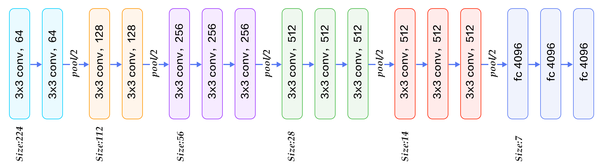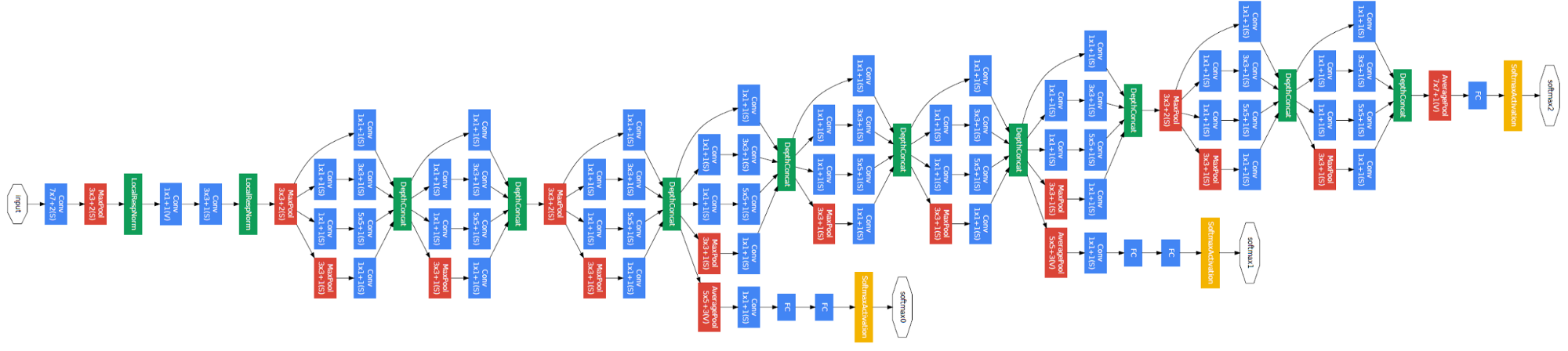×

Search anything:

# Feed Forward Neural Networks

#### Machine Learning (ML) artificial neural networks neural network feed forward neural networkFREE BOOK -> Problems for the day before your Coding Interview (on Amazon)

A feedforward neural network is an Artificial Neural Network in which connections between the nodes do not form a cycle. The feedforward neural network was the first and simplest type of artificial neural network. In this network, the information moves in only one direction, forward, from the input nodes, through the hidden node and to the output nodes.It does not form a cycle.

In feed Forward Neural Networks:

• Inputs are fed by a series of weights which is then computed by Hidden Layers
• The hidden layers job is to transform the inputs into something that the output layer can use
• Hidden Layers use Activation Function to maps the resulting Values in 0 or 1.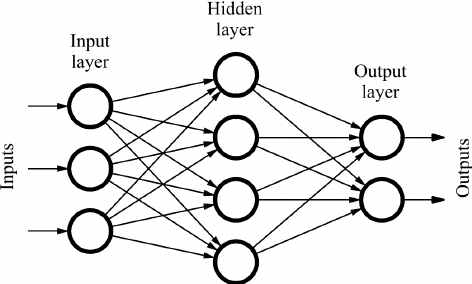Hidden Layers Mostly uses RELU Activation Function. In RELU activation Function:

if x is less than 0 the Output becomes 0 and if it is greater than 0 It Becomes x to determine the output of neural network like yes or no.

Activation Function maps the resulting values in between 0 to 1 or -1 to 1 etc. (depending upon the function). Again the Output from the hidden layers are then feed into the Output Layer.

The Output layer Uses Softmax Activation Function to map values to find the probabilities of the Outputs.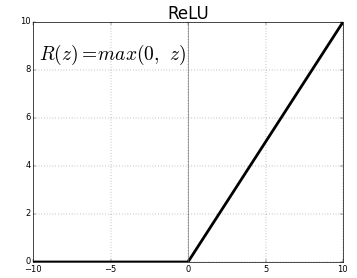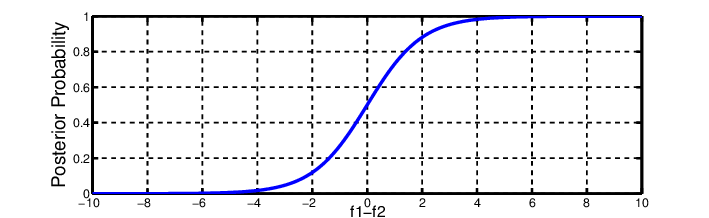When we have Multi Layers Perceptron, each weight is updated by backpropagation.

Here the output values are compared with correct answer to compute the value of error function. The error function we mostly used is Gradient Descent.

In Gradient Descent, we minimized cost Function by trial and error by just trying lots of values and visually inspecting the resulting graph.then It calculates the proper weights of the Input values and they are again feed into the Input layer. After repeating this process for a sufficiently large number of training cycles, the network will usually converge to some state where the error of the calculations is small.There may be the case of Overfitting where the model fits too well with the training data but does not perform too well with test data. We use Early Stopping to prevent overfitting. We use Multiple Hidden layers to improve model performance but it takes much time.

### Types Of Neural Networks

#### Feedback ANN

1. Feedback ANN – In these type of ANN, the output goes back into the network to achieve the best-evolved results internally. The feedback network feeds information back into itself and is well suited to solve optimization problems. Feedback ANNs are used by the Internal system error corrections.

#### Feed Forward ANN

1. Feed Forward ANN – A feed-forward network is a simple neural network consisting of an input layer, an output layer and one or more layers of neurons.Through evaluation of its output by reviewing its input, the power of the network can be noticed base on group behavior of the connected neurons and the output is decided. The main advantage of this network is that it learns to evaluate and recognize input patterns.

#### Classification-Prediction ANN

1. Classification-Prediction ANN –It is the subset of feed-forward ANN and the classification-prediction ANN is applied to data-mining scenarios. The network is trained to identify particular patterns and classify them into specific groups and then further classify them into “novel patterns” which are new to the network.

### Most Commonly Used Neural Networks

In Deep Learning are Convolution Neural Networks (CNN) and Recurrent Neural Networks.

CNN is used in computer vision like object Detection like YOLO and SSD and Image detection. GANs are very popular to improve the quality of the Image in Computer Vision.

There are Several Architectures of CNN like:

### AlexNet

1.Alexnet It has 7 hidden layers 1 Input Layer and 1 Output Layer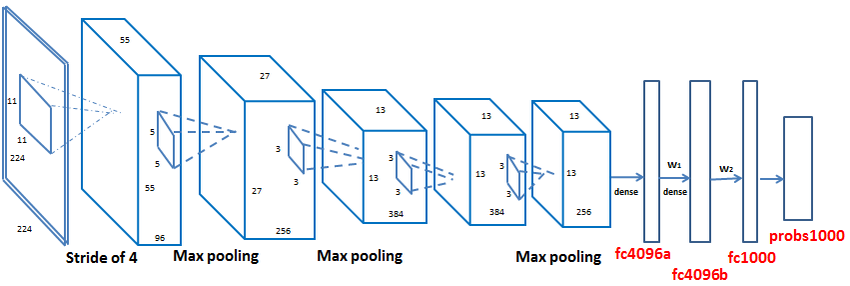### VGG19

2.VGG has 19 hidden layers 1 Input and 1 Output layer